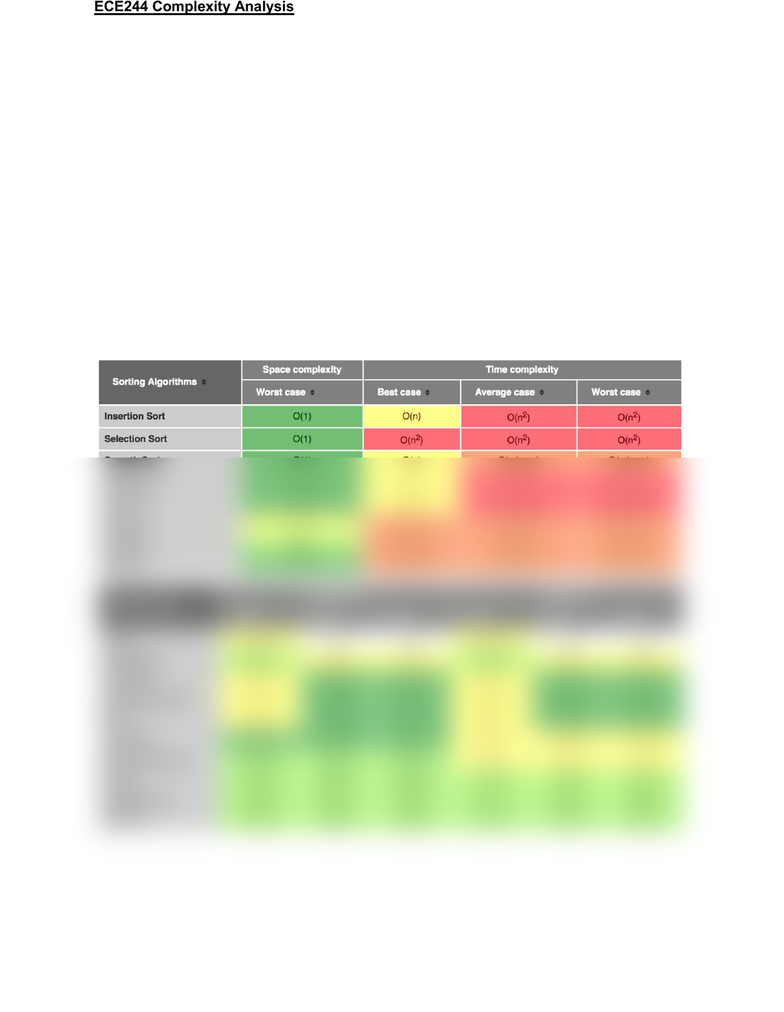Textbook Notes (280,000)
CA (170,000)
UTSG (10,000)
ECE (80)
Chapter 18

# ECE244H1 Chapter 18: ECE244 Complexity Analysis

Department
Electrical and Computer Engineering
Course Code
ECE244H1
Professor
Tarek Abdelrahman
Chapter
18

This preview shows half of the first page. to view the full 2 pages of the document.ECE244 Complexity Analysis
Execution Time depends on:
1. hardware/compiler/etc (machine)
2. size of the input (n)
3. input itself (data in the array in relation to t)
4. “Steps” executed by the program (algorithm)
Step → instruction/operation/code fragment/etc that takes a constant amount of time (i.e., an
amount of time independent of the input size and the actual data) on any machine
Time = f(machine, n, data) < sec > → Time = f(n, data) < steps >
What are the 3 scenarios for input data?
1. Best case: input data causes the algorithm to execute the least number of steps
2. Worst case: input data causes the algorithm to execute the largest number of steps
3. Average: input data causes the algorithm to execute an “average” number of steps